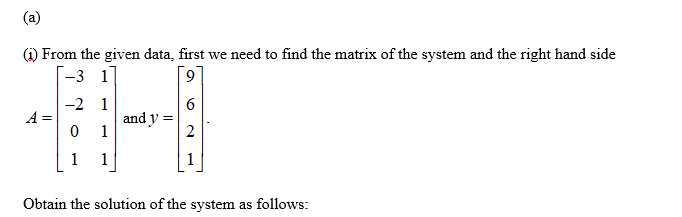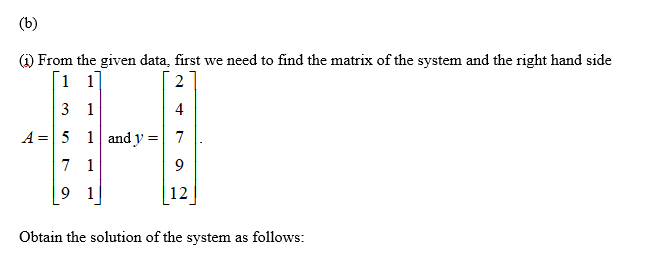# For each of the sets of data that follows, use the least squares approximation to find the best fits with both (i) a linear function and (ii) a quadratic function. Compute the error E in both cases.(a) {(-3, 9), (−2, 6), (0, 2),(1, 1)}(b) {(1, 2), (3,4), (5, 7), (7, 9), (9, 12)}(c) {(−2, 4), (−1, 3), (0, 1), (1,−1), (2,−3)}

Question
1 views

For each of the sets of data that follows, use the least squares approximation to find the best fits with both (i) a linear function and (ii) a quadratic function. Compute the error E in both cases.

(a) {(-3, 9), (−2, 6), (0, 2),(1, 1)}

(b) {(1, 2), (3,4), (5, 7), (7, 9), (9, 12)}

(c) {(−2, 4), (−1, 3), (0, 1), (1,−1), (2,−3)}

check_circle

Step 1Step 2

(ii) From the given data, the right hand side stays the same, but for quadratic approximation, we are adding another column in A:

Step 3...

### Want to see the full answer?

See Solution

#### Want to see this answer and more?

Solutions are written by subject experts who are available 24/7. Questions are typically answered within 1 hour.*

See Solution
*Response times may vary by subject and question.
Tagged in

### Math